Sunday , November 29 2020# Motion and Time: 7th Class NCERT CBSE Science Ch 13

## Motion and Time 7th Class NCERT CBSE Science Chapter 13

#### Question: Give the basic unit of speed.

Answer: Metre / second is the basic unit of speed.

#### Motion and Time NCERT – Question: Mention which is the most common thing in almost all the clocks.

Answer: The most common thing in almost all the clocks is that all of them shows periodic motion.

#### Question: Paheli and Boojho have to cover different distances to reach their school but they take the same time to reach the school. What can you say about their speed?

Answer: They do not have equal speed because they cover unequal distance in equal intervals of time. One of them has higher speed whom has to cover larger distance with respect to other.

#### Question: If Boojho covers a certain distance in one hour and Paheli covers the same distance in two hours, who travels with a higher speed?

Answer: Boojho travels with a higher speed as he has covered same distance in lesser time with respect to Paheli.

#### Question: A simple pendulum is oscillating between two points A and B as shown in figure. Is the motion of the bob uniform or non-uniform?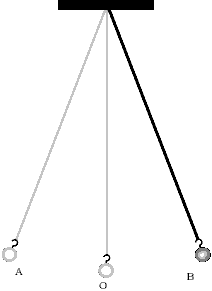Answer: The motion of bob is non-uniform as it does not cover equal distance in equal intervals of time.

#### Question: State the characteristic of distance-time graph for the motion of an object moving with a constant speed.

Answer: The distance-time graph for the motion of an object moving with a constant speed is a straight line inclined on x-axis at some angles.

#### Question: Name the axis that represents dependent variable.

Answer: y-axis represents the dependent variable.

#### Question: Briefly explain how will decide which object is moving fast and which one is moving slow.

Answer: If an object covers more distance in equal intervals of time with respect to other, then we can say that object is moving faster with respect to the other object.

#### Question: If you did not have a clock, how would you decide what time of the day is?

Answer: We can decide time of the day without clock by seeing shadow formed by the sun, e.g. at noon, shadow formed by the sun is shorter than at evening.

#### Motion and Time NCERT – Question: State how do we measure time interval of a month.

Answer: Time interval of a month is measured by one new moon to the next.

#### Question: Determine the number of seconds there in a day.

In a day, we have 24 h
We know that 1 h = 3600 s
So, 24 h = 3600 × 24 = 86400s

#### Question: Estimate that how many hours are there in a year.

In a year, we have 365 days and 1 day = 24 h
So, for 365 days, we have 24 × 365 h = 8760 h

#### Question: Explain how time was measured when pendulum clocks were not available.

Answer: Before the discovery of pendulum clocks, sundials, water clocks and sand clocks were used.

#### Question: A spaceship travels 36000 km in one hour.Express its speed in km/s.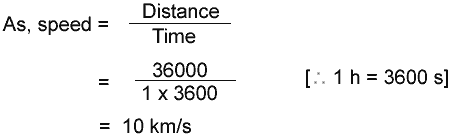#### Question: Out of the two given clocks, a pendulum clock or a quartz clock. Mention which of the clock between the given ones requires an electric cell for its working?

Answer: A quartz clock requires an electric cell for its working.

#### Question: Name the most ancient unit of time in which it was earlier measured.

Answer: Solar day is the most ancient unit of time in which it was earlier measured.

#### Question: State the condition under which the distance-time graph of an object is a horizontal line parallel to the axis of time.

Answer: When the object is at rest, then the distance-time graph of an object is a horizontal line parallel to the axis of time.

#### Question: State the factors on which the time period of a simple pendulum depend.

Answer: The factors on which the time period of a simple pendulum depend are

1. Length of the pendulum
2. Acceleration due to gravity.

#### Question: Name the different types of graphs.

Answer: Different types of graphs are

1. Line graph
2. Pie chart
3. Bar graph

#### Question: State the way through which we can prepare a pendulum by our own.

Answer: By suspending a metal ball with the help of a cotton thread and the other end of the thread can be tied to some different supports, so in this way, we can prepare a pendulum by our own.

#### Then, she thought to go through her own car. So, while moving she increased the speed of the car from 36 km/h to 54 km/h. All of sudden, a rickshaw came in front of her car but due to applying of brakes at the right time, both of them got safe from meeting with an accident.

1. Calculate the increase in the speed of the car in the terms of m/s.
2. Mention a positive action performed by Priyanka here.

1. Initial speed = 36 km/h = 5/18 × 36 = 10m/s
Final speed = 54 km/h = 5/18 × 54 = 15 m/s
∴ Increase in speed = Final speed – Initial speed = (15-10) m/s = 5 m/s
2. Priyanka applied the brakes at a right time while driving the car and prevented her family and the person on rickshaw from meeting with an accident.

#### Question: Differentiate between a uniform and non-uniform motion.

Answer: When a body travels equal distance in an equal interval of time, then the body is said to be in a uniform motion.

When a body travels unequal distance in an equal interval of time, then the body is said to be in non-uniform motion.

#### Question: Briefly mention how many types of motion are there. Also, name all of them.

Answer: Different types of motion are there and they can be named as follows

1. Periodic motion
2. Rolling motion
3. Rotatory motion
4. Oscillatory and vibratory motions
5. Translatory motion

#### Question: The average age of children of class VII is 12 years and 3 months. Express this age in second.

Answer: Given, the average age of children 12 years and 3 months
1 year = 365 days = 365 × 24 h [1 day = 24 h] = 365 × 24 × 3600 s [1 h = 3600 s] 12 years = 31536000 s × 12 = 378432000 s
3 months = 30x3days = 30 × 3 × 24h = 30 × 3 × 24 × 3600s = 7776000s
So, total age in second = 378432000 + 7776000 = 386208000s

#### Motion and Time NCERT – Question: Plot a distance-time graph of the tip of the second hand of a clock by selecting 4 points on X-axis and Y-axis, respectively. The circumference of the circle traced by the second hand is 64 cm.

 Time (s) X 15 30 45 60 Distance (cm) Y 16 32 48 64

As, here equal distance is covered in equal intervals of time.
So, graph will be straight line.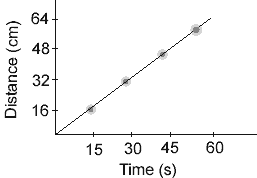#### Question: With the help of the data given in the following table, show graphically the relationship between the diameter and circumference of a circle.

 Diameter of the circle (cm) Circumference of the circle (cm) 1 3.2 2 6.3 3 9.4 4 12.6 5 15.7 6 18.8

Answer: With the help of a given data in a tabular form, the graph can be obtained by taking diameter of the circle on the x-axis and the circumference on y-axis. On x-axis, 1 cm diameter is shown by 1 cm and on the y-axis, 3 cm of circumference is shown by 1 cm.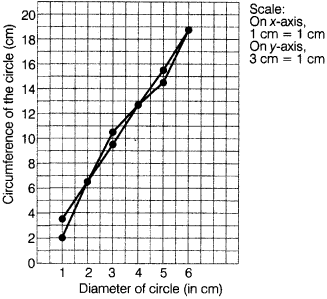#### Question: Starting from A, Paheli moves along a rectangular path ABCD as shown in figure. She takes 2 min to travel each side. Plot a distance-time graph and explain whether the motion is uniform or non-uniform.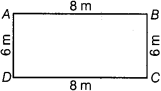Answer: Since, the distance covered per unit time for the entire distance covered is not the same, the motion is a non-uniform motion.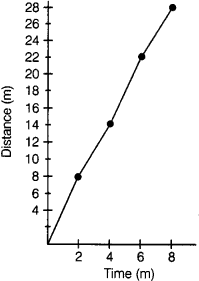#### Question: One day, a five years old boy, Chintoo was playing with his toys in his room. After few minutes, he saw a wristwatch on his study table, so after seeing it, he got crazy and so much in eager to play with that watch. Then, for 2 to 3 min, he was trying to analyse it that whether its a toy or something else, then suddenly, he was just about to throw it but at the same his mother entered into the room and take the watch back immediately from his hand.

1. State the benefit of a wristwatch.
2. Briefly mention the values showed by Chintoo’s mother here.

1. The wristwatch can be handled very easily to check the time in comparison to the wall clock.
2. The values showed by Chintoo’s mother here is the concern towards her four years old child and also the concern towards that wristwatch while considering the importance of money.

#### Question: The following distance-time graph of three objects (D, E and F) are given (see figure given alongside). What can you say about the motion of the objects?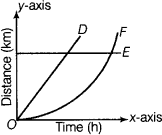Answer: Since, the distance-time graph of an object D is a straight line therefore, its motion is uniform. Also here, we can see that the distance-time graph of object E is parallel to x-axis (time-axis), therefore object E is at rest.
As, the distance-time graph of object F is a curved line, therefore the object C is moving with a non-uniform speed and its motion is non-uniform.

#### Motion and Time NCERT – Question: The following figure shows the distance-time graph for the motion of two objects D and E. Which one of them is moving slower?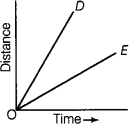Answer: After studying the figure given alongside, D or E is moving slower as the slope of distance-time graph of E is less than that of graph D.

#### Question: The following figure shows the distance-time graph for the motion of two vehicles (C and D). At what time and distance (from the starting point), they will cross each other.

Answer: Firstly, draw perpendicular lines from point E on x-axis and y-axis.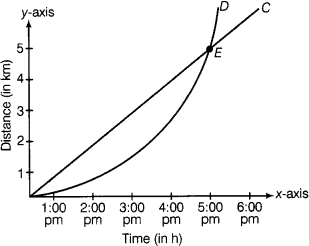The two vehicles (C and D) will meet (or cross) each other at 5:00 PM and after travelling a distance of 4 km.

#### Question 1. Given below as a figure is the distance-time graph of the motion of

1. What will be the position of the object at 20 s?
2. What will be the distance travelled by the object in 12 s?
3. What is the average speed of the object?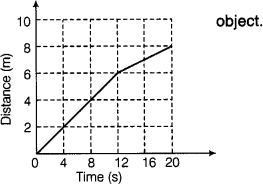1. From the graph, it is clear that the distance at 20 s is 8 m.
2. Distance travelled by the body object in 12 s is 6 m.
3. As, average speed =#### Question: Boojho goes to the football ground to play football. The distance-time graph of his journey from his home to the ground is given as figure.

1. What does the graph between points B and C indicate about the motion of Boojho?
2. Is the motion between 0 to 4 min uniform or non-uniform?
3. What is his speed between 8 to 12 min of his journey?

1. Since, the graph between B and C is parallel to time-axis, so it indicates that Boojho is at rest.
2. Since, the graph is not straight line, so it is a non-uniform motion.
3. As, speed=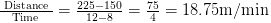#### Question: Explain by giving example that how can we choose a suitable scale.

Answer: While choosing the most suitable scale for drawing a graph, we should keep the following points in mind

1. The difference between the highest and lowest values of each quantity.
2. The intermediate values of each quantity so that with the scale chosen, it is easy to mark the values on the graph.
3. To utilize the maximum part of the paper on which the graph is to be drawn, assume that we have a graph paper of size 25 cm x 25 cm and we have to accommodate following data:
 Time (AM) Odometer reading Distance from the starting point 8:00 AM 36540 km 0 km 8:30 AM 36560 km 20 km 9:00 AM 36580 km 40 km 9:30 AM 36600 km 60 km 10:00 AM 36620 km 80 km

Since, one of the scales will be
Distance 5 km = 1 cm and Time 6 min = 1 cm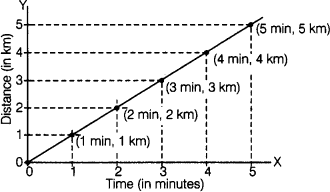#### Question: Distance between Bholu’s and Golu’s house is 9 km. Bholu has to attend Golu’s birthday party at 7 O’ clock. He started his journey from his home at 6 O’ clock on his bicycle and covered a distance of 6 km in 40 min. At that point, he met Chintu and he spoke to him for 5 min and reached Golu’s birthday party at 7 O’ clock. With what speed, did he cover the second part of the journey? Calculate-his average speed for the entire journey.

Answer: From the question, Bholu covers 3 km distance in 15 min.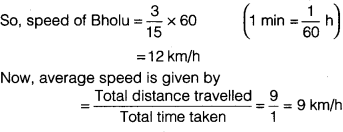#### Question: The given data regarding the motion of two different objects C and D is in a tabular form. Check them carefully and state whether the motion of the objects is uniform or non-uniform.

 Time Distance travelled by C (in m) Distance travelled by D (in m) 9:30 AM 10 12 9:45 AM 20 19 10:00 AM 30 23 10:15 AM 40 35 10:30 AM 50 37 10:45 AM 60 41 11:00 AM 70 44

Answer: We can check the motion of the objects C and D by plotting the distance-time graph for the two objects.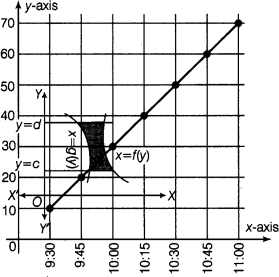Graph the object C on x-axis
Scale 1 cm = 15 min and on y-axis 1 cm = 10 m
Since, the distance-time graph of the object C is a straight line. Therefore, its motion is uniform.
Graph of object D
Scale On x-axis, 1 cm = 15 min, On y-axis 1 cm = 5mSince, the distance-time graph of the object D is not a straight line, therefore, its motion is non-uniform.

### Fill in the Blanks – Motion and Time NCERT:

1. The distance covered by an object in a ……………….. is called its speed.
2. Time between one sunrise and the next sunrise was called a ……………….. .
3. In a simple pendulum, the metallic ball suspended by thread is called its ……………….. .
4. Time period of a pendulum depends on its ……………….. .
5. The symbols of all units are written in ……………….. .
6. A ……………….. is one billionth of a second.
7. Motion of objects can be represented in the pictorial form by their ……………….. graph.
8. The distance-time graph for the motion of an object moving with a constant speed is a ………………..

1. unit time
2. day
3. bob
4. length
5. singular
6. nanosecond
7. distance-time
8. straight line

### True / False

1. The motion of a spinning top is rotational motion.
2. The smallest time interval that can be measured with commonly available clocks and watches is one second.
3. The time period of simple pendulum is not constant.
4. Faster vehicle has a higher speed.
5. All the clocks make use of some periodic motion.
6. The basic unit of speed is km/h.
7. The symbols of all units are written in singular.
8. The basic unit of time is second.
9. The distance-time graph of standing vehicle is a straight line parallel to x-axis.
10. Periodic events are used for the measurement of time.

1. True
2. True
3. True
4. True
5. True
6. False, the basic unit of speed is m/s
7. True
8. True
9. True
10. True

#### Motion and Time NCERT – Question: Match the Column I with Column II.

 Column I Column II (a) Years (i) rotation period of the earth on its axis (b) Hour (ii) time to complete 100 m race (c) Minute (iii) gestation period in humans (d) Days (iv) age of a person (e) Second (v) in scientific research (f) Month (vi) time to reach your school (g) Microseconds (vii) train reaches from one city to other

1. (a)-(iv)
2. (b)-(viii)
3. (c)-(vi)
4. (d)-(i)
5. (e)-(ii)
6. (f)-(iii)
7. (g)-(v)

## Understanding Media: NCERT 7 Class CBSE SST Civics 06

Understanding Media: NCERT 7th CBSE Social Studies Civics Chapter 06 Question: What is called ‘media’? Answer: Everything …